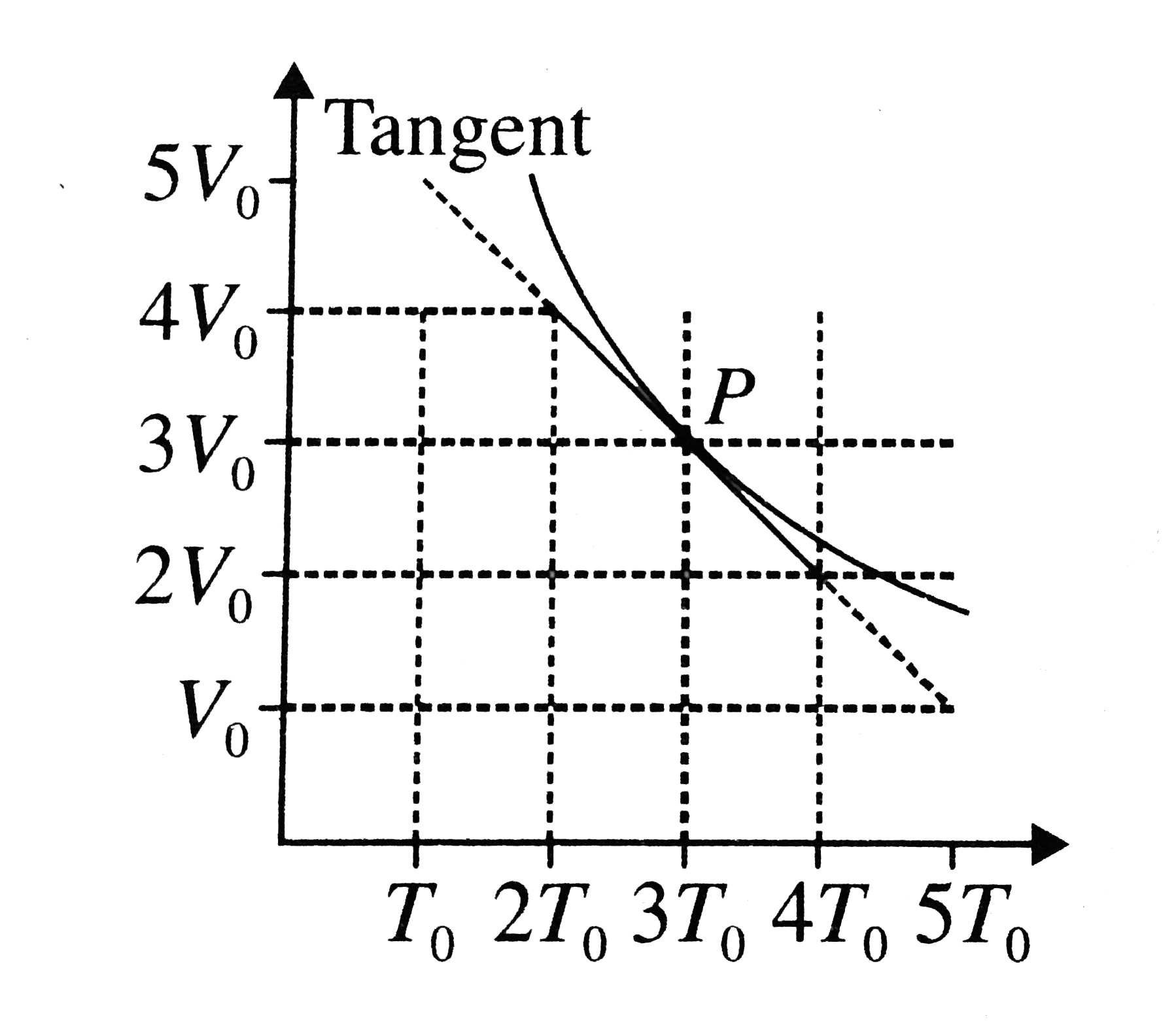Doubtnut App - Get 50 Lakh+ video solutions better than GoogleInstall Now

# Figure shows the adiabatic curve for n moles of an ideal gas, the bulk modulus for the gas corresponding to the point P will beA

5nRT03V0

B

nR(2+T0V0)

C

nR(1+T0V0)

D

2nRT0V0

Text Solution
Verified by Experts

## d. For adiabatic process : Bulk modulus : B=γP for point p:P=nRTV=nR3T03V0=nRT0V0 ⇒B=γnRT0V0 Now TVγ−1= constant ⇒(γ−1)TdV+VdT=0 ⇒dVdT=−V(γ−1)T for point P→ −3V03T0=−(3V0)(γ−1)(eT0)0 ⇒γ=2 so from Eq. (i) B=2nRT0V0